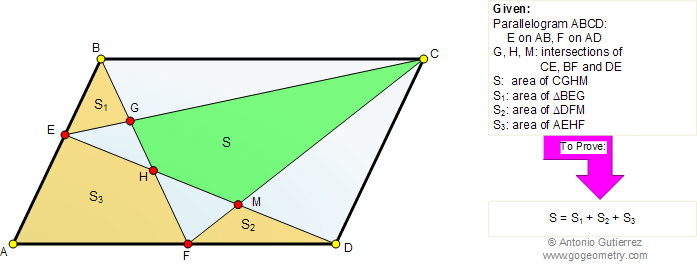# Online Geometry Problem 477: Parallelogram, Triangle, Quadrilateral, Area

The figure shows a parallelogram ABCD. Lines BF and CF intersect DE at H and M, respectively. Lines BF and CE meet at G. If S, S1, S2, and S3, are the areas of quadrilateral CGHM, triangle BEG, triangle DFM, and quadrilateral AEHF, respectively, prove that S = S1+ S2 + S3.Home | Geometry | Search | Problems | All Problems | 471-480 | Area of a Parallelogram | Email | Solution / comment | By Antonio Gutierrez